# Brilliant Mechanics Contest - Season 1

Welcome all to the first ever Brilliant Mechanics Contest. Like the Brilliant Integration Contest, the aim of the Mechanics Contest is to improve skills and techniques often used in Olympiad/JEE-style Mechanics problems. But above all, the main reason is so we can have fun.

Anyone is allowed to participate, as long as they adhere to the following rules.

1. I will post the first problem. If someone solves it, he or she can post a solution and then must post a new problem.

2. A solution must be posted below the thread of the problem. Then, the solver must post a new problem as a separate thread.

3. Please make a substantial comment.

4. Make sure you know how to solve your own problem before posting it, in case no one else is able to solve it within 48 hours. Then, you must post the solution and you have the right to post a new problem.

5. If the one who solves the last problem does not post a new problem in 24 hours, the creator of the previous problem has the right to post another problem.

6. The scope of the problems is (IPhO) Olympiad Mechanics.

7. Keep yourself restricted to Newtonian Mechanics. Do not use Lagrangian mechanics

8. You are allowed to apply a slight dressing of Electricity And Magnetism to your Mechanics Problem.

9. It is NOT compulsory to post original problems. But make sure it has not been posted on brilliant.

10. Try to post the simplest solution possible. For example, if someone posted a solution using Newton's Laws, when there is a solution using only Work Energy theorem, the latter is preferred.

11. There should be only one question. No sub questions allowed.No parts. Only one answer should be there.

12. The problem creator should give the answer to his/her question with the question.

13. Post only the problem and it's solution here. Extremely relevant comments are allowed. All discussions should be done here

Format your proof as follows:

Solution to Problem (Insert Problem no here)

[Post your solution here]

And put your Problem in a new thread following the format :

Problem (Insert Problem no here)

[Post your problem here]

Remember to reshare this note so it goes to everyone out there. And above all else, have fun!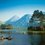Note by Rajdeep Dhingra
5 years, 5 months ago

This discussion board is a place to discuss our Daily Challenges and the math and science related to those challenges. Explanations are more than just a solution — they should explain the steps and thinking strategies that you used to obtain the solution. Comments should further the discussion of math and science.

When posting on Brilliant:

• Use the emojis to react to an explanation, whether you're congratulating a job well done , or just really confused .
• Ask specific questions about the challenge or the steps in somebody's explanation. Well-posed questions can add a lot to the discussion, but posting "I don't understand!" doesn't help anyone.
• Try to contribute something new to the discussion, whether it is an extension, generalization or other idea related to the challenge.
• Stay on topic — we're all here to learn more about math and science, not to hear about your favorite get-rich-quick scheme or current world events.

MarkdownAppears as
*italics* or _italics_ italics
**bold** or __bold__ bold
- bulleted- list
• bulleted
• list
1. numbered2. list
1. numbered
2. list
Note: you must add a full line of space before and after lists for them to show up correctly
paragraph 1paragraph 2

paragraph 1

paragraph 2

[example link](https://brilliant.org)example link
> This is a quote
This is a quote
    # I indented these lines
# 4 spaces, and now they show
# up as a code block.

print "hello world"
# I indented these lines
# 4 spaces, and now they show
# up as a code block.

print "hello world"
MathAppears as
Remember to wrap math in $$ ... $$ or $ ... $ to ensure proper formatting.
2 \times 3 $2 \times 3$
2^{34} $2^{34}$
a_{i-1} $a_{i-1}$
\frac{2}{3} $\frac{2}{3}$
\sqrt{2} $\sqrt{2}$
\sum_{i=1}^3 $\sum_{i=1}^3$
\sin \theta $\sin \theta$
\boxed{123} $\boxed{123}$

## Comments

Sort by:

Top Newest

Problem 21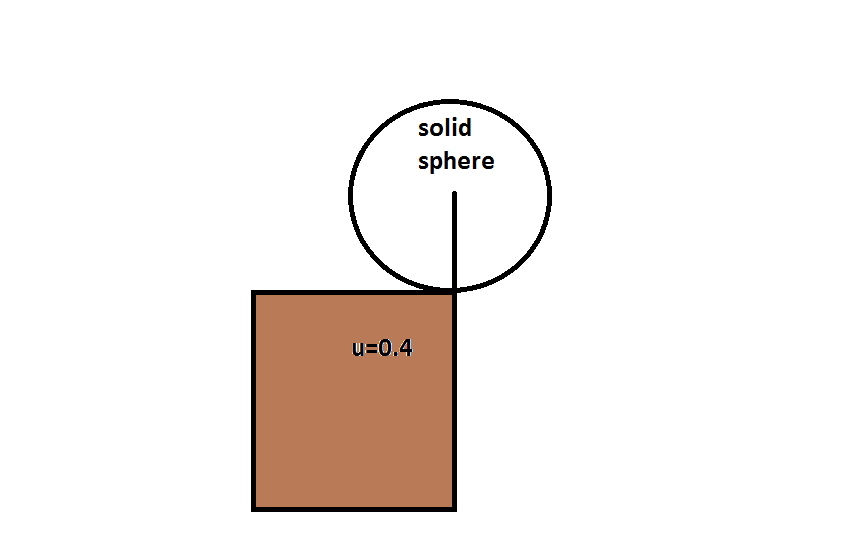A solid spherical ball is placed carefully on the edge of a table in the position shown in the figure. The coefficient of static friction between the ball and the edge of the table is 0.4 . It is then given a very slight push. It begins to fall off the table.

Find the angle (in degrees) turned by the ball before it slips.

Archit Agrawal has solved it along with a nice solution

- 5 years, 3 months ago

Log in to reply

Solution to Problem 21: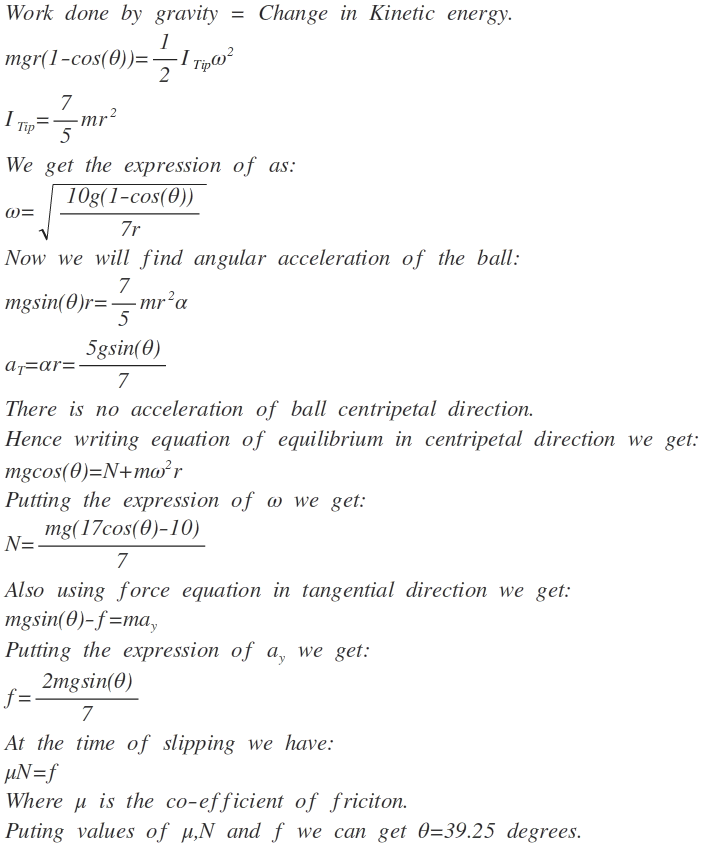Insight Provided by Aryan Goyat

Nice solution , but i would like to add one thing to people who were getting around 19.33 as answer they were actually missing the thing that the the ball is doing pure rolling and would slip when max friction is just causing rolling and immediately after this this slipping begins .so take it analogical to sphere rolling on ground and then look for relation between f and mgsin(Q).The approach that f =mgsin(Q).is wrong.if the sphere had not been rolling then the point of contact would have already changed as the particles would have followed center of mass including the bottommost point.imagine the above case without friction i hope you got your mistake.

- 5 years, 3 months ago

Log in to reply

Problem 9:fluid-mech The system is placed as shown above. The fluid inside is water.The system is initially at rest. The radius of three semicircular arcs is $\dfrac{h}{\pi}$. The system is released now. Find the acceleration acting on particle at point $P$ when the difference between the levels becomes $2h$.

Edit: Assume that the column is of uniform cross section.

Aditya Kumar has solved this problem with the nice solution.

- 5 years, 5 months ago

Log in to reply

Solution to Problem 9:

This question is going to be solve using Conservation of Energy:

Let $\rho$ be the density of the liquid and A be the area of cross section of the column.

Change in Potential Energy in left column=$A\rho gx$=Change in Potential Energy in right column.

Therefore, total change in potential energy=$2A\rho gx$

Change in potential energy = Change in kinetic energy

$2A\rho gx$=$\frac{13\rho Axv^2}{2}$

Therefore, $v^2=\frac{4gx}{13}$ ............(1)

On differentiating both sides wrt time "t",

We get tangential acceleration $a_{t}=\frac{2g}{13}$

For Centripetal acceleration $a_{c}=\frac{6\pi}{13}$

We got this by using the formula: $a_{c}=\frac{v^2}{r}$

Therefore resultant acceleration is: $a=\frac { 2g }{ 13 } \sqrt { { 9\pi }^{ 2 }+1 }$

This question is solved by me by using a small hint from a friend.

- 5 years, 5 months ago

Log in to reply

Problem 1

Consider the following figure.A is a sleeve which is fitted on a thick Rigid rod. One end of the rod is connected with a light rigid rod of length l. Another identical light rigid rod has its one end fixed to A. Both the rods are connected by a pivot C which in turns hangs a block X. We start moving A to the right with a velocity $v_0$ which is kept constant. Let the angle made by the Thick rod and the Light Rod ,attached to the thick rod's end, at any instant be $\theta$. Find the velocity of block X (which is same as pivot C's translational Velocity ) as a $f^n$ of $\theta$.

Hint: $f^n$ means Function. $\ddot\smile$.

Satyendra Kumar has solved it along with the nice solution.

- 5 years, 5 months ago

Log in to reply

$\text{Solution to Problem 1:}$$x=l\cos\theta\Longrightarrow\dfrac{dx}{dt}=-l\sin\theta\times\dfrac{d\theta}{dt},similarly\\ y=l\sin\theta \Longrightarrow \dfrac{dy}{dt}=l\cos\theta\times\dfrac{d\theta}{dt},also\\ v=\dfrac{d}{dt}(2l\cos\theta) i.e\ v=-2l\sin\theta\times\dfrac{d\theta}{dt}\ using\ the\ above\ equations\ we\ get,\\ horizontal\ velocity\ of\ point\ C=\dfrac{v}{2}\\ vertical\ velocity\ of\ point\ C=\dfrac{v\cot\theta}{2}\\ \therefore\ velocity\ of\ point\ C=\dfrac{v\csc\theta}{2}$ $\text{Problem 2:}$ A particle executes SHM of period $7.5$ seconds.The velocity of a point $P$ $1m$ away from the centre of motion is $2ms^{-1}$.Find the amplitude of the oscillations.If the particle is passing through $P$ and moving away from the centre of oscillation,find the time that elapses before the particle next passes through $P$. $\text{Answer is A=2.6m,time=2.8s}$.

- 5 years, 5 months ago

Log in to reply

How the time is 2.8s?

Can you please add an explanation?

- 5 years, 5 months ago

Log in to replyFirst we draw the generating circle for the SHM.Let $t$ be the time for the particle to go from point P to the extreme end and back to P.Using $x=a \times \cos \theta$ we get,$1=2.6 \cos\dfrac{\omega t}{2}$,this gives us $t=2.8s$.

- 5 years, 5 months ago

Log in to reply

$\textbf{Problem 11:}$Consider the system given in the figure(All surfaces are smooth). The block of mass M collides elastically with the first of the two blocks connected by a spring of stiffness $k$ with speed $v$. Your job is to find the condition on mass $M$ such that , at any instant $t$, it collides with the first block again.

By condition I mean the value of M in terms of the known constants.

Samarpit Swain has posted the solution himself in case no one else posted it within the time restriction.

- 5 years, 5 months ago

Log in to reply

$\textbf{Solution to problem 11:}$

Let velocity of $M$ be $v_{M}$ and $m$(first block) be $v_{m}$ after the first collision. By conservation of linear momentum and definition of $e$,we have:

$v_{M}= \dfrac{(M-m)v}{(M+m)}= \dfrac{(1-\phi)v}{(1+\phi)}$ $v_{m}=\dfrac{2Mv}{M+m}=\dfrac{2v}{1+\phi}$

where $\phi=\dfrac{m}{M}$

Now the for the second collision at any instant $t$ the position vectors(w.r.t ground frame) of $M$ and $m$ must be equal at that instant. If the collision is considered along the x-axis, then:

$x_{M}=v_{M}t= \dfrac{(1-\phi)vt}{(1+\phi)}$

Furthermore,The blocks connected to the spring will be executing simple harmonic motion with angular frequency $\omega=\sqrt{\frac{2k}{m}}$. Let the equation of SHM be $x=A\sin(\omega t)$. Since the CoM of the spring mass system would moving with a constant velocity of $v_{c}=\dfrac{v_{m}}{2}=\dfrac{v}{1+\phi}$, we have:

$x_{c} = \dfrac{vt}{1+\phi}$

$\omega A= \dfrac{v}{1+\phi}$

$\therefore A= \dfrac{v}{(1+\phi)\omega}$

Shifting back to ground frame, we have:

$x_{m} =x_{c}+A\sin(\omega t)= \dfrac{vt}{1+\phi}\left(1+\dfrac{\sin(\omega t)}{\omega t}\right)$

For second collision $x_{M}=x_{m}$

$\therefore M=\dfrac{-m\omega t}{\sin(\omega t)}$

- 5 years, 5 months ago

Log in to reply

Problem 13

A small body slides down an inclined surface passing into a loop from the minimum height ensuring that the body does not leave the surface of the loop. What symmetrical segment with an angle $\alpha < 90^{\circ}$ can be cut out of the loop for the body to reach point B after travelling a certain distance in the air ? How will the body move if $\alpha$ is greater or smaller than the found one ? Disregard friction and air resistance.###### This Problem is NOT Original.

Aditya Kumar has solved it along with a nice solution

- 5 years, 5 months ago

Log in to reply

Solution to Problem 13:Image

I took minimum for h because there is a minimum height required for having motion around a complete circle.

- 5 years, 5 months ago

Log in to reply

Problem 14:

A chimney of length l initially stands upright. It is given a tiny kick, so that it topples over. At what point along its length is it most likely to break? Assume that the chimney consists of boards stacked on top of each other, and that each board is attached to the two adjacent ones with tiny rods at each end.Image

Rajdeep Dhingra has solved it along with a nice Solution

- 5 years, 5 months ago

Log in to reply

Solution to Problem 14 :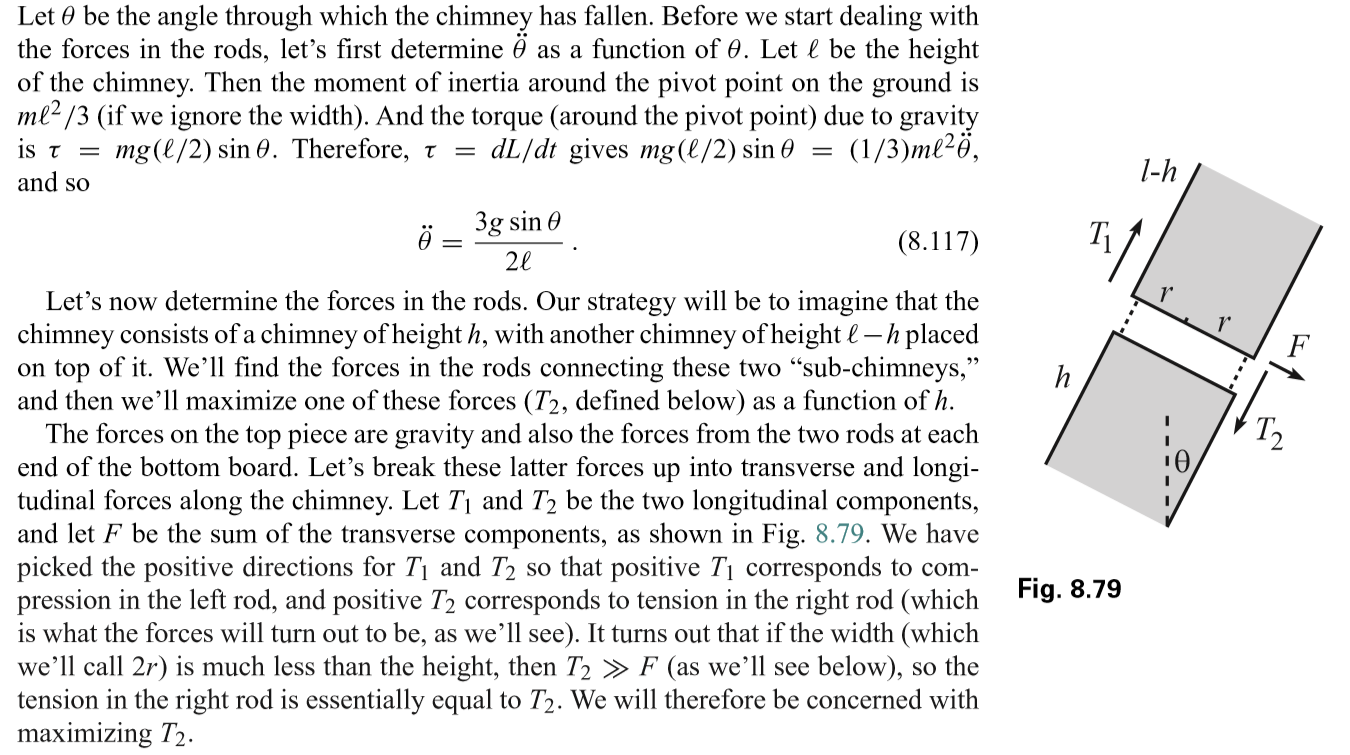Page 1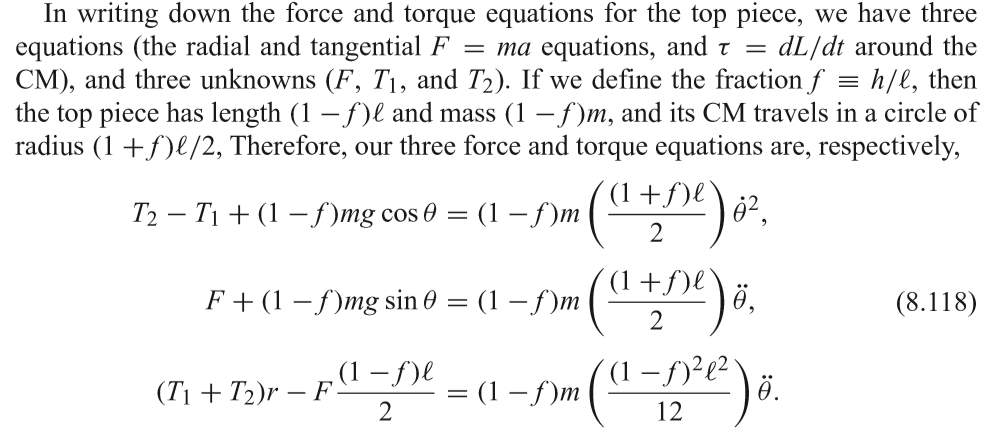Page 2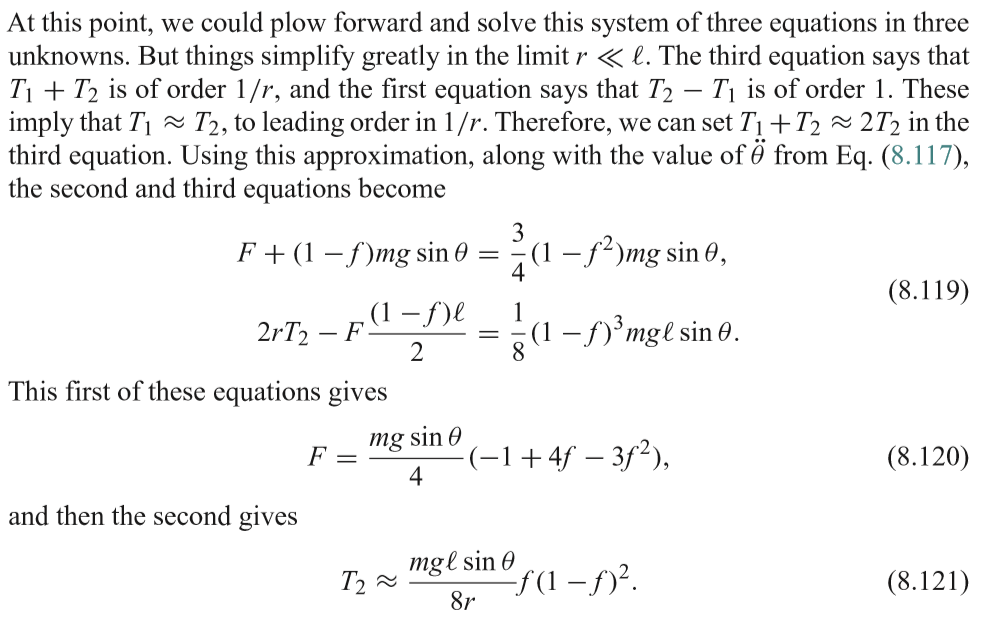Page 3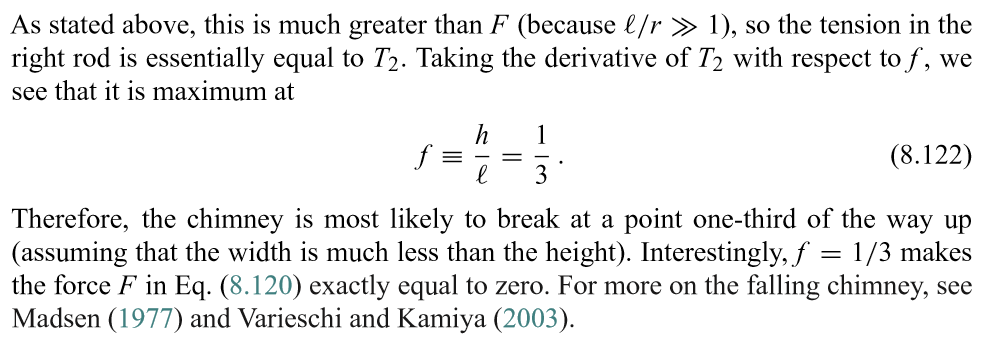Page 4

- 5 years, 4 months ago

Log in to reply

Problem 18:

A steel ball of diameter $d=3.0 mm$ starts sinking with zero initial velocity in a sample of olive oil whose viscosity is $\eta =0.9P$.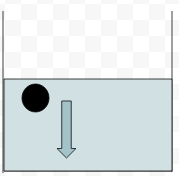Simultaneously, a spring is placed in a gravity free environment with a damping coefficient of $\beta =10{ s }^{ -1 }$.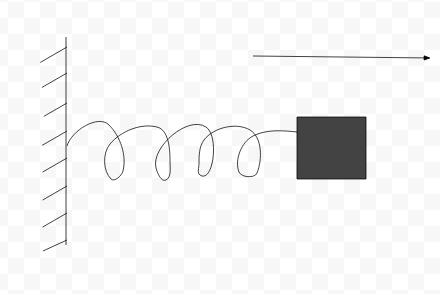By the time the ball reaches $99 \%$ of the steady state velocity in the liquid, find the change in the amplitude of the spring's motion, given that it's initial amplitude was $20m$.

Density of steel = $8050Kg/{ m }^{ 3 }$. Ignore the force of up-thrust by the liquid.

Vighnesh Shenoy has solved it along with a great solution

- 5 years, 3 months ago

Log in to reply

Solution to Problem 18 :

The only forces acting on the body are it's weight $mg$ downwards and the viscous force upwards $F_{visc} = 6\pi \eta r v$ according to stoke's law.

The steady state velocity $v_{t}$ is obtained when these forces are balanced.

$6\pi \eta r v_{t} = mg \rightarrow 6\pi \eta r (0.99v_{t}) = 0.99mg$

Now, it's acceleration at any instant of time is given by,

$a = \dfrac{mg-6\pi \eta r v}{m}$

$\therefore \dfrac{dv}{dt} = \dfrac{mg-6\pi \eta r v}{m}$

$\displaystyle \int_{0}^{0.99v_{t}} \dfrac{m}{mg-6\pi \eta r v}dv = \displaystyle \int_{0}^{t} dt$

Integrating,

$\dfrac{m}{6\pi \eta r v} \left[\ln(mg - 6\pi \eta r v)\right]_{0.99v_{t}}^{0} = t$

$t = \dfrac{m}{6\pi \eta r }\cdot \ln \left(\dfrac{mg}{mg-6\pi \eta r (0.99v_{t})}\right) = \dfrac{m}{6 \pi \eta r } \cdot \ln \left( \dfrac{1}{0.01}\right) = \dfrac{m}{6 \pi \eta r } \ln(100)$

$t = \dfrac{m}{6\pi \eta r }\ln(100)$

Putting $m = \rho \dfrac{4 \pi r^{3} }{3}$

$t = \dfrac{2\rho\ln(100)r^{2}}{9\eta} = \dfrac{\rho\ln(100)d^{2}}{18\eta}$

The amplitude at any time t is given by ,

$A(t) = A_{0}e^{-\beta t}$

$\Delta A = A_{0}\left[1 - e^{\dfrac{\rho \beta d^{2} \ln(100)}{18\eta}} \right ]$

Plugging in the values we get,

$\Delta A \approx 17.4497 m$

- 5 years, 3 months ago

Log in to reply

Problem 5

A Smooth light horizontal rod AB Can rotate about a vertical axis passing through its end A . the rod is fitted with a small sleeve of mass m attached to the end A by a weightless spring of length l and stiffness K . what work must be performed to slowly get this system going and reaching the angular velocity w .

Abhineet Nayyar has solved it along with the nice solution.

- 5 years, 5 months ago

Log in to reply

Solution to Problem 5:

For the final position of the sleeve, net outward force = net inward force $Kx=M(l+x){ \omega }^{ 2 }$

Solving this, $x=\frac { Ml{ \omega }^{ 2 } }{ K-M{ \omega }^{ 2 } } \quad and\quad l+x=\frac { Kl }{ K-M{ \omega }^{ 2 } }$

Now total work done will be stored as the sum of elastic potential energy of the spring and the rotational kinetic energy of the sleeve, so,

$\quad \quad \quad W=\frac { 1 }{ 2 } I{ \omega }^{ 2 }+\frac { 1 }{ 2 } K{ x }^{ 2 }\\ \Rightarrow \quad W=\frac { 1 }{ 2 } M{ (l+x) }^{ 2 }{ \omega }^{ 2 }+\frac { 1 }{ 2 } K{ x }^{ 2 }$

Substituting the initially calculated values and taking some terms common,

$W=\frac { 1 }{ 2 } \frac { { l }^{ 2 }KM{ \omega }^{ 2 } }{ { (K-M{ \omega }^{ 2 }) }^{ 2 } } (K+M{ \omega }^{ 2 })$

- 5 years, 5 months ago

Log in to reply

Problem 8: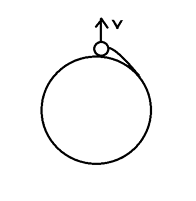A thread is wound around a fixed cylinder with its free end tied to a sphere so that the sphere touches the cylinder. Subsequently the sphere attains a radial velocity $v$ away from the cylinder and the thread starts unwinding.

Determine the length $l$ of the unwound segment of thread at time $t$. Surya Prakash has solved the problem.

- 5 years, 5 months ago

Log in to reply

Solution To Problem 8 :pic

Let at any instant the length of unwinded string be $x$. Let it now rotate through an angle $d \theta$. Assume that $d \theta \rightarrow 0$. Then, we can make the approximation as shown in figure. So, from figure, $dx= R d \theta$. But now as $dx$ is very small, we can take that $\dfrac{d \theta}{dt} = \dfrac{v}{x}$.

So,

$xdx = 2Rv \, dt \\ \dfrac{x^2 }{2} = 2Rvt + c$

It is clear that from initial conditions $c=0$.

Therefore, $\boxed{l=\sqrt{2Rvt}}$.

- 5 years, 5 months ago

Log in to reply

Problem 19

A rigid rod of mass $(m)$, and length $(L)$ is kept with one of its ends at height $(h)$ above the ground as shown. The rod intially makes an angle $( \beta )$ with the horizontal. The rod is allowed to fall freely. If the co-efficient of restitution of the ground is equal to $e$ find magnitude of difference between the angular velocity and velocity of center of mass of rod after collision.

Details and Assumptions
- $m = 2 kg$
- $L = 1 m$
- $h = 5 m$
- $\beta = \dfrac{\pi}{3} rad$
- $e = \dfrac{4}{5}$
- $g = 10 \dfrac{m}{s^{2}}$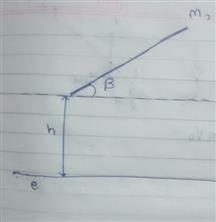Aryan Goyat has solved it with a nice solution

- 5 years, 3 months ago

Log in to reply

Solution to Problem 19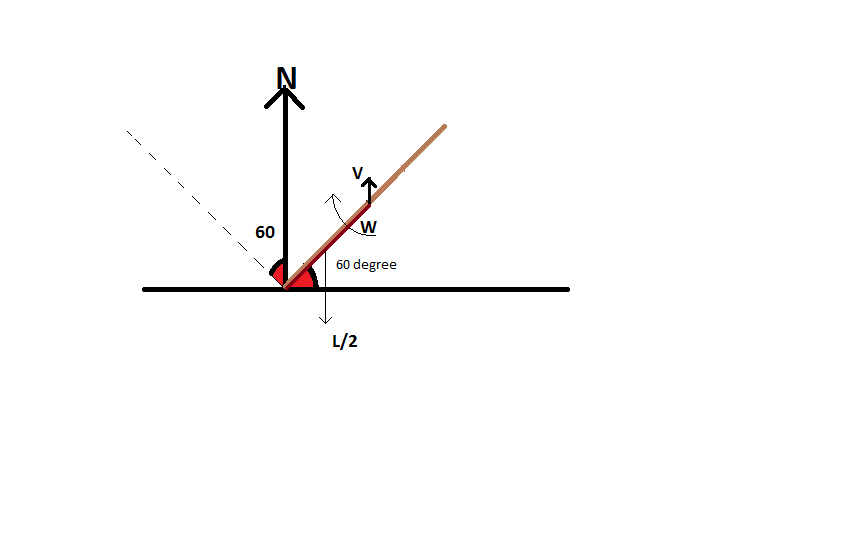Let the reaction due to collision be N, velocity of the centre of mass after collision $v$,angular velocity afterwards be $\omega$. let the time of contact during collision be $t$. Now since $t$ is very very small, the net change brought by gravity during this time interval can be neglected.

Velocity of rod just before collision(u) = $\sqrt { 2gh } =10m/s$

Also, $N \times t = m(v+u$

$N\times\frac {L}{2} \times Cos(60) = \frac { M{ L }^{ 2 }\omega }{ 12t }$

$v+\omega \frac { L }{ 2 } cos(60)\quad =\quad e\times 10$

Solving above equations, we get $v=\frac {2}{7}$ and $\omega= \frac {216}{7}$

- 5 years, 3 months ago

Log in to reply

Problem 20

A regular polygon with perimeter of 144m and n>3 has balls kept on all its vertices.there is one ball of mass 4kg and rest balls have a mass 2kg.The ball of mass 4kg is given a velocity v and it strikes a neighbouring ball of mass 2 kg and after deflection(elastic collision) it strikes the next ball and so on reaches back to its original position.What is the maximum circumradius of polygon possible.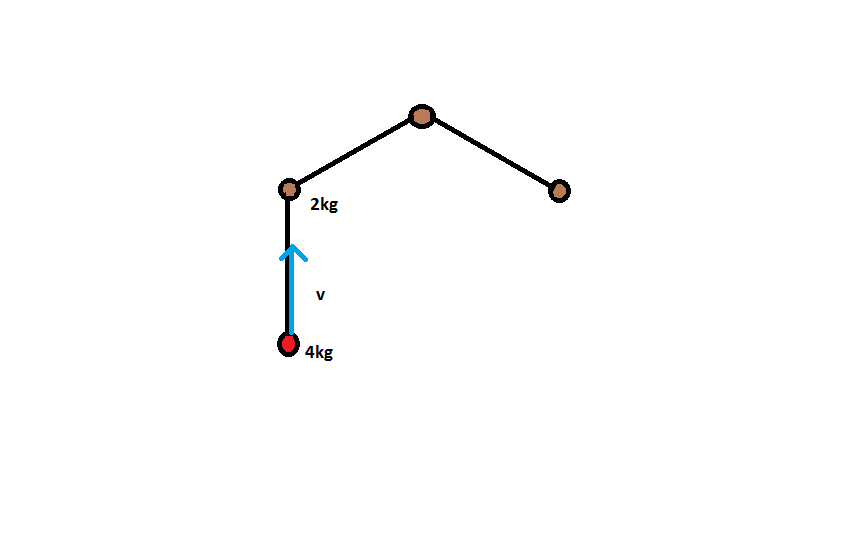- 5 years, 3 months ago

Log in to reply

Solution to Problem 20:

Consider the following figure before the collision. Let the mass of the target be $m$. So, the mass of the projectile is $2m$. The initial velocity of the projectile is $v$ whereas it's velocity after the collision is $v'$. Consider the angle between the two directions of the projectile as $\theta$. Also, take the velocity of the target along the initial direction, as ${ u }_{//}$ and that perpendicular to the initial direction as ${ u }_{ \bot }$.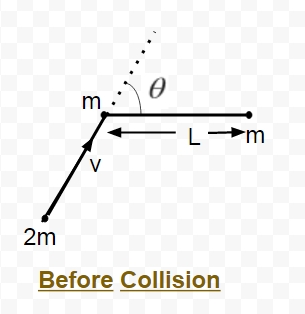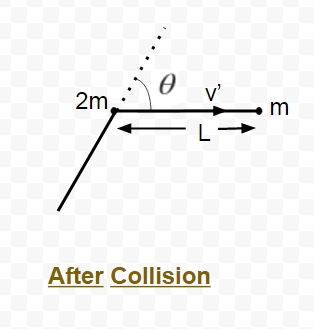(i) Applying principle of momentum conservation along the initial direction of motion of projectile:

$2mv = m{ u }_{//} + 2mv'\cos { \theta }$

So, by rearranging the terms:

${ u }_{//} = 2v-2v'\cos { \theta }$ $\quad \quad \quad--------- 1.$

(ii) Now, applying momentum conservation perpendicular to the initial direction of motion of projectile:

$0 = m{ u }_{ \bot } - 2mv'\sin { \theta }$

which gives us:

${u}_{ \bot } = 2v'\sin { \theta }$ $\quad \quad \quad-----------2.$

Now, using conservation of kinetic energy before and after the collision:

$\frac { 1 }{ 2 } (2m){ v }^{ 2 }=\frac { 1 }{ 2 } m{ u }^{ 2 }+\frac { 1 }{ 2 } (2m){ v' }^{ 2 }$

Now, substituting ${ u }^{ 2 }={ { u }_{ // } }^{ 2 }+{ { u }_{ \bot } }^{ 2 }$ and using the respective values from $1.$ and $2.$:

$2{ v }^{ 2 }=6{ v' }^{ 2 }+4{ v }^{ 2 }-8(v)(v')\cos { \theta }$ OR

$3{ v' }^{ 2 }-4(v)(v')\cos { \theta +{ v }^{ 2 } } =0$

For the projectile to continue on it's subsequent path, the above mentioned quadratic equation should have real roots, So:

$16{ v }^{ 2 }\cos ^{ 2 }{ \theta } -12{ v }^{ 2 }\ge 0$ OR

$\cos { \theta } \ge \frac { \sqrt { 3 } }{ 2 }$

Also, from the geometry of an n-sided polygon, we know that the angle theta is given by $\frac { 2\pi } { n }$.

So, $0\le \frac { 2\pi }{ n } \le \frac { \pi }{ 6 }$ which further implies $12\le n\le \infty$

Therefore, limiting value of $n$ = $12$, and hence, it's circumradius:

$R = \frac { L }{ 2\sin { \frac { \pi }{ n } } } =\frac { \frac { Perimeter }{ n } }{ 2\sin { \frac { \pi }{ n } } }$

Plugging in the values, we get:

$R = \frac { \frac { 144 }{ 12 } }{ 2\sin { \frac { \pi }{ 12 } } } =\frac { 6 }{ \sin { \frac { \pi }{ 12 } } } =23.1822m$

- 5 years, 3 months ago

Log in to reply

I hope this is right!! :P

Is the answer $6/sin(\pi/12)$ = $23.1822m$?

- 5 years, 3 months ago

Log in to reply

Problem 4In the figure shown, a solid sphere of mass m and radius r is released from a height 6r to slide down a smooth surface.A plank of same mass 'm' touches the horizontal portion of the surface at the ground.The coefficient of friction between plank and sphere is $\mu$ and that between the plank and the ground is $\frac{\mu}{4}$.

Find the work done by the friction force between the plank and the ground till sphere starts pure rolling on the plank. Neglect height of the plank.It is not original.

6r is the height of centre of sphere from plank

Prakhar Bindal has solved it along with the nice solution.

- 5 years, 5 months ago

Log in to reply

Solution to Problem 4:

Call the velocity of sphere before reaching the plank to be v .

Now i will work from the reference frame of ground .

Till pure rolling starts frictional force umg will act on the sphere in backward direction and due to third law the same force will act on the plank in forward direction .

For the plank there will be two frictional forces acting on it . the one acting in forward direction will have magnitude umg and the one acting in backward direction will have magnitude u/4 * 2mg = umg/2

let after time t pure rolling starts.

writing equations of motion for the sphere and plank .

Vs = v-ugt

Vp = ugt/2

writing torque equation about centre of mass of sphere we get

Angular acceleration = 5ug/2R

w = 5ugt/2r

For pure rolling Vs - Rw = Vp

Solving t= v/4ug .

work done by frictional force = umg/2 * displacement of plank

displacement of plank = 1/2 * ug/2 * (time)^2

Solving this we get required answer

- 5 years, 5 months ago

Log in to reply

Problem 23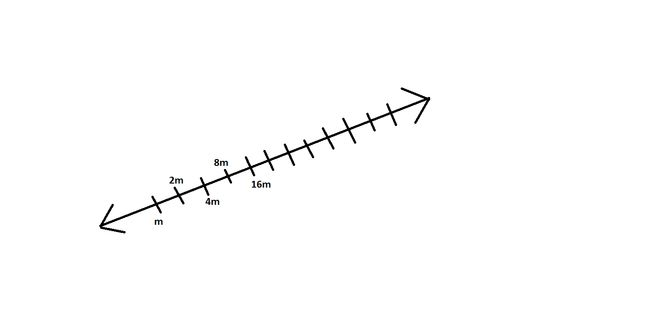Consider a number line at x we put a mass $\large 2^x m \text{ for } x \geq 1$. We first give a velocity to mass at x=1 towards right side of number line and then a chain of collision stats we find the distance between the balls at x=100 and x=101 when ball at 101 and 102 collide and report it as p. Find $\displaystyle \large f{\left(\frac{100055!}{p}\right)}$ where f(y) is a function such that it is the sum of digits in y and then the number obtained and so on until we get the single digit number. For eg:- $f(125600)=f(1+2+5+6+0+0)=f(14)=f(1+4)=5$.
Consider all collision to be elastic.

Harsh Shrivastava has solved it with a nice solution

- 5 years, 3 months ago

Log in to reply

Solution to problem 23:

(I will use $x$ for a ball of mass $2^{x}m$.)

Since collisions are elastic:

(i)coefficient of restitution = 1

(ii)velocity of approach == velocity of separation

(iii)momentum remains conserved.

Let the velocity given to the first ball be $v$.

Now using (iii), $2mv = 2mv_{1} + 4mv_{2}$ , where $v_{1} \rightarrow \text{velocity of first ball after collision}$ and $v_{2} \rightarrow \text{velocity of second ball after collision.}$

Also , using (ii), $v = v_{1} + 2v_{2}$

Solving these two equations, we get $v_{1} = -v/3 ; v_{2} = 2v/3$

[Negative sign shows that after collision, first ball will move in backward direction.]

Similarly, velocity of 3rd ball after collision with second ball will be $4v/9$.

Thus proceeding as above,velocity of ball of mass $2^{x}m$ after collision with (x-1)th ball will be $(\frac{2}{3})^{x-1} v$.

Now velocity of ball 100 after collision with ball 99 will be $\frac{2^{99}}{3^{99}} v$.

Now the ball 100 will collide with ball 101.

Using (ii) and (iii), velocity of ball 101 after collision will be $k$ where $k =\frac{2^{100}}{3^{100}} v$

and velocity of ball 100 be t , $t = \frac{-2^{99}}{3^{100}} v$.

Distance between each ball $= 1 \text{unit}$.

Time taken by ball 101 to reach ball 102 = $\dfrac{1}{k}$

Distance travelled by ball 100 in backward direction in this time = $\dfrac{t}{k}$.

Thus distance between ball 100 and ball 101 $= 1-(-\dfrac{t}{k})$ which on simplifying gives $1.5$ units.

Thus we need to find out value of $f(2a/3)$ where a is that giant number.

Note that f(q) returns q if q is a multiple of 9.

Clearly 9 divides 2a/3.

Thus f(2a/3) = 9.

- 5 years, 3 months ago

Log in to reply

Problem 24

A cat sitting in a field suddenly sees a standing dog. To save its life, the cat runs away in a straight line with speed u. Without any delay, the dog starts with running with constant speed v>u to catch the cat. Initially, v is perpendicular to u and L is the initial separation between the two. If the dog always changes its direction so that it is always heading directly at the cat, find the time the dog takes to catch the cat in terms of v, u and L.

Surya Prakash solved it along with a nice solution

Question shared with me by @Rakesh Ramachandiran.

- 5 years, 3 months ago

Log in to reply- 5 years, 3 months ago

Log in to reply

Irodov problem 13

- 2 years ago

Log in to reply

Try to find the radial acceleration of the dog wrt ground at that moment.

- 2 years ago

Log in to reply

Problem 3:Image

A tablecloth covers a horizontal table and a steel ball lies on top of it. The tablecloth is pulled from under the ball, and friction causes the ball to move and roll. Prove that at the moment when the ball reaches a stage of just before pure rolling it stops translating.

Assume that the table and table cloth is infinitely long.

Gautam Sharma has solved it along with the nice solution.

- 5 years, 5 months ago

Log in to reply

Solution to problem 3 At pure rolling let speed of table cloth slipping be $v$ So $v$ will be speed of centre of mass in left direction.

Also it will be rotating with angular velocity$= \omega$ in anticlockwise.

Also $v=r \omega$

About point of contact centre of mass will have velocity=$r \omega$ due right.

So total speed of centre of mass will be

$v_{cm}=v-r \omega$

But $v=r \omega$ .Hence $v_{cm}=0$

- 5 years, 5 months ago

Log in to reply

PROBLEM 6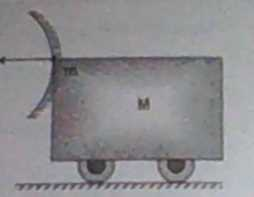A tank of mass $M$ fires a bomb of mass $m$ with a horizontal velocity $v$ relative to the tank. The tank is fitted with a concave mirror of focal length $f$ facing towards the receding bomb (as shown in the figure).

Find the Magnitude of the velocity of the image with respect to the object, when bomb is at a distance of $R/4$ from the mirror, where $R$ is the radius of curvature of the mirror.

Neglect gravity

Rajdeep Dhingra has solved it along with the nice solution.

- 5 years, 5 months ago

Log in to reply

Solution to Problem 6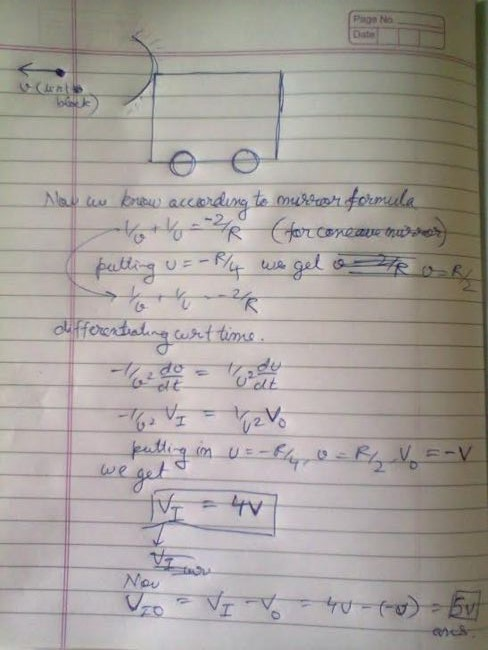- 5 years, 5 months ago

Log in to reply

Problem 7Two blocks A and B are kept on a smooth surface. Block A has a mass $m_1$ and block B has a mass $m_2$. They are attached by a light spring of natural length l and stiffness k. A force F of constant magnitude is applied on B towards the right. Find the maximum elongation in the spring.

Ishan Dasgupta Samarendra has solved it along with the nice solution.

- 5 years, 5 months ago

Log in to reply

$\dfrac{2m_1F}{k(m_1+m_2)}?$

- 5 years, 5 months ago

Log in to reply

 $\textbf{Solution to Problem 7}$  Working from the Center of Mass frame, consider the system '$2 blocks + spring$'. The CM has an acceleration of $\frac{F}{m+M}$. Consequently, on both blocks, a pseudo force of $Ma$ and $ma$ respectively has to be applied towards the left.

Thus, on the right block, there is a net external force of $F_2=F-M\frac{F}{m+M}=\frac{mF}{m+M}$ while on the left block there is a net external force of $F_1=\frac{mF}{m+m}$. 

From the CM it will appear as though the blocks move away from each other until at the instant of maximum elongation they come to instantaneous rest. 

Let the displacement of the left block from the CM be $x_1$ to the left and the right one, $x_2$ to the right. 

From a modified version of the Work Energy Theorem, 

$W_{NC}+W_{ext}=\Delta TE$

$F_1x_1+F_2x_2=\dfrac{1}{2}k(x_1+x_2)^2$

- 5 years, 5 months ago

Log in to reply

Problem 10:

A ball with uniform mass density rolls without slipping on a turntable. If the frequency of the turntable is f find the frequency of the ball.

Hint: First try to show that the ball moves in a circle (as viewed from the inertial lab frame).

Samarpit Swain has solved it along with a nice solution

- 5 years, 5 months ago

Log in to reply

$\textbf{Solution to Problem 10:}$

Let the axis of rotation of the turntable pass through the origin and the plane of rotation be the x-y plane. At any instant let the position vector of the of the ball be $\vec{r}$. Let R be its radius.

Also the pure rolling motion is caused by the frictional force $f$. Call the angular velocity of the disc as $\vec{\omega_{t}}$ and for the ball, $\vec{\omega_{b}}$. The equations of general motion of the ball are given by:

$f =m\frac { dv }{ dt } ............(1)$(translational motion) $-{ f }\times R =I\frac { d\omega_{b} }{ dt } ........(2)$(rotational motion)

Now, define the velocity $\vec{v}$ of ball in lab frame. This is simply the vector sum of velocity of disc(at $\vec{r}$) and the velocity of ball.

$\therefore \vec{v}= \vec{\omega_{t}}\times \vec{r} + \vec{\omega_{b}}\times R.......(3)$

Differentiating Eq(3) w.r.t to time, we get: $\frac { dv }{ dt } =\omega_{t} \times \frac { dr }{ dt } +\frac { d\omega_{b} }{ dt } \times R \\ \\$

Now, work out the cross product of the above equation.Clearly the only unknown now left is $\frac { d\omega_{b} }{ dt }$. So combine Equation 1 and 2, then substitute it in Equation 3. Finally we get:$\frac { dv }{ dt } =\omega_{t} \times v-\left( \frac { m{ R }^{ 2 } }{ I } \right) \frac { dv }{ dt }$

Now rearranging and plugging the value of $I$ we get: $\vec{a}=\left( \frac { 2 }{ 7 } \omega_{t}\right)\times v$

This equation describes the trajectory of a particle moving in circular motion and the ratio of their frequencies comes out to be $2:7$

- 5 years, 5 months ago

Log in to reply

Problem 17 :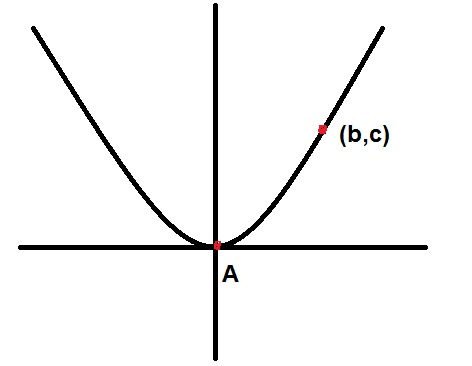A ball of mass '$m$' and radius '$r$' rolls without slipping on a parabolic path starting from rest from the point $(b,c)$. Find the force exerted by the ball on the surface as it passes through the point $A$. Assume that $r << b,c$.

Abhineet Nayyar has solved it along with a nice solution

- 5 years, 4 months ago

Log in to reply

Solution to Problem 17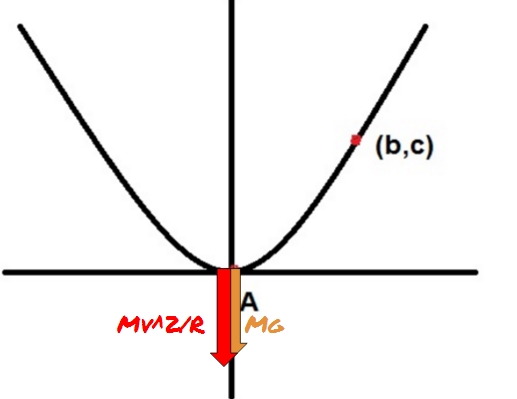So, clearly, it can be seen from the diagram, that when the body reaches the point $'A'$, it will have it's weight i.e. $mg$ acting downwards. Along with this, since the body would have acquired a velocity $v$, hence it would also feel centrifugal force that would tend to press on the frame and hence add on to it's weight. All we need to do is to calculate this force.

By applying energy conservation, Loss in potential energy = Gain in kinetic energy,

So, $\frac { 1 }{ 2 } m{ v }^{ 2 }(1+\frac { 2{ r }^{ 2 } }{ 5{ r }^{ 2 } } =mgc\\ \Rightarrow v=\sqrt { \frac { 10 }{ 7 } gc }$.

Also, since the centrifugal force is equal to the centripetal force, and for that we need a radius. Now, since this curve is not exactly a circle, so we use the concept of Radius of curvature. Let that be given by $'r'$.

Formula for the radius of curvature is given by $r=\frac { { (1+{ (y' })^{ 2 }) }^{ \frac { 3 }{ 2 } } }{ y'' }$

Here, $y=\frac { { x }^{ 2 } }{ 4a }$, $y'=\frac { x }{ 2a }$, $y''=\frac { 1 }{ 2a }$ and $a$ is the focal length of the given parabola. Putting these values in the formula for $r$ and substituting $x=0$ for point $'A'$, we get $r=2a$.

Now, for calculating $a$, we use the fact that $(b,c)$ lie on the curve. Hence, ${ c }^{ 2 }=4ab\\ \Rightarrow \quad a=\frac { { c }^{ 2 } }{ 4b }$.

Hence, we get $r=\frac { { c }^{ 2 } }{ 2b }$.

Finally, centrifugal force is given by ${ F }_{ c }=\frac { m{ v }^{ 2 } }{ r } =\frac { m(\frac { 10gc }{ 7 } ) }{ \frac { { b }^{ 2 } }{ 2c } } =\frac { 20mg{ c }^{ 2 } }{ 7{ b }^{ 2 } }$.

Therefore, total force = ${ F }_{ c }+mg=\frac { 20mg{ c }^{ 2 } }{ 7{ b }^{ 2 } } +mg$

:)

- 5 years, 4 months ago

Log in to reply

Problem 22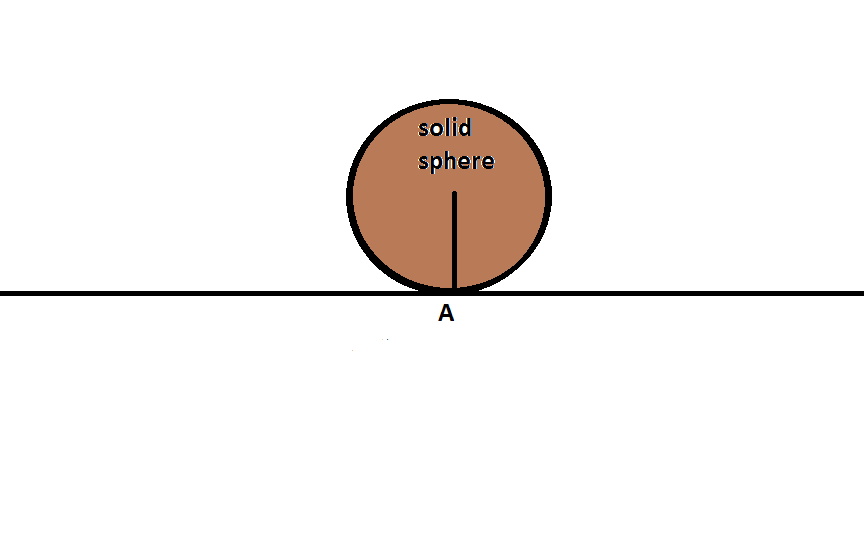A sphere is doing pure rolling with constant $\omega =6.2831\text{ rad/s }$. What is the distance travelled by the it bottom-most point in 1s?

Take $r=6.2831 \text{ m}$.

Archit Agrawal has posted it along with a nice solution.

- 5 years, 3 months ago

Log in to reply

Answer to problem 22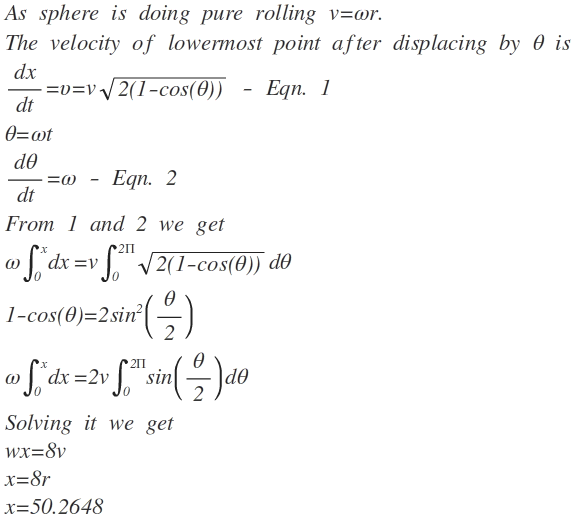dx is small displacement.

- 5 years, 3 months ago

Log in to reply

Problem 25

N balls are constrained to move in one dimension. If you are allowed to pick the initial velocities, what is the maximum number of collisions you can arrange for the balls to have among themselves?

Assume the collisions are elastic.

- 5 years, 3 months ago

Log in to reply

@CH Nikhil I have solved this question.is the answer (n)(n-1)/2

- 4 years, 1 month ago

Log in to reply

Are the balls identical?

- 5 years, 3 months ago

Log in to reply

They are identical. They are allowed to move infinitely. The spacing does not matter since time is not involved.

- 5 years, 3 months ago

Log in to reply

Are they all at rest initially ? do we velocity to only one of them ?

- 5 years, 3 months ago

Log in to reply

we can assign velocities to all of them.

- 5 years, 3 months ago

Log in to reply

He got the question from A book "introduction to classical mechanics"

- 5 years, 3 months ago

Log in to reply

Who is the author ?

- 5 years, 3 months ago

Log in to reply

Just Google it

- 5 years, 3 months ago

Log in to reply

Thanks. I found $\huge \ddot \smile$

- 5 years, 3 months ago

Log in to reply

Are the balls identical ? Are they allowed to move infinitely ? Are they spaced equally ?

- 5 years, 3 months ago

Log in to reply

Ques of David morin

- 2 years, 4 months ago

Log in to reply

Problem 2: (Proposed by Satyendra Kumar)

A particle executes SHM of period $7.5$ seconds.The velocity of a point $P=1m$ away from the centre of motion is $2ms^{-1}$ .Find the amplitude of the oscillations.If the particle is passing through $P$ and moving away from the centre of oscillation, find the time that elapses before the particle next passes through $P$.

Aditya Kumar has solved it along with the nice solution.

- 5 years, 5 months ago

Log in to reply

Solution to Problem 2:

Time period, T=7.5s

Therefore, angular velocity $\omega$=$\frac{2\pi}{T}$=$0.8377s^{-1}$

Velocity of the particle performing SHM at any position is given by: ${ v }_{ x }^{ 1}=\omega(A^2-x^2)^{\frac{1}{2}}$

where x is the distance from centre.

Therefore, amplitude, A=2.588m

- 5 years, 5 months ago

Log in to reply

Aditya, we undoubtedly know $v_x=\omega \sqrt{\text{A}^2-x^2}$. But you wrote $v_x^2=\omega (\text{A}^2-x^2)^{\frac{1}{2}}$. Most probably that's why the answer is not corresponding with Rajdeep's answer. Calculator says $\sqrt{(\frac{15}{2\pi})^2+1}=2.588$

Please check it!

- 5 years, 5 months ago

Log in to reply

$\textbf{Problem 12:}$

Consider a cylindrical chamber(length $2l$) containing an ideal gas which follows the law $PV^{m}=c$. A piston of mass $M$ ,uniform cross-sectional area $A$ and negligible thickness divides the chamber in two equal partitions containing gas of equal pressure $P$ and volume $V$.Now the piston is slightly displaced on one side. Will the system perform SHM? If yes, find the resulting time period for small oscillations.

Rajdeep Dhingra has solved it along with a Nice solution

- 5 years, 5 months ago

Log in to reply

Solution to Problem 12

Let the Piston move a small distance x. So, the Volume and Pressure slightly changes on both sides, causing a pressure difference, generating a force. Now, $PV^m = c \\ \text{Differentiating we get} \\ V^mdP + mV^{m-1}PdV = 0 \\ \Rightarrow \frac{dP}{P} = \frac{-mdV}{V} \\ dV = Ax , V = Al \\ \Rightarrow dP = \frac{-mxP}{l}$ Since Pressure changes both sides with opposite signs the Pressure Difference , $\Delta P$, will be $\Delta P = \frac{-2mP}{l}x = \frac{F}{A} \\ F = -\frac{2mPA}{l}x = Ma \\ a = -\frac{2mPA}{Ml}x \\ \Rightarrow \omega^2 = \frac{2mPA}{Ml} \\ \Rightarrow T = \boxed{2\pi\sqrt{\frac{Ml}{2mPA}} }$

Is it correct @Samarpit Swain ?

- 5 years, 5 months ago

Log in to reply

Problem 15 :

A homogeneous rod AB is lying on a perfectly smooth floor. A bullet hits the rod and gets stuck in it. The direction of the bullet's initial velocity $v_0$ is perpendicular to the rod, and the point where the bullet hits lies at a distance x from the middle of the rod. The mass of the bullet is m and the mass of the rod is M.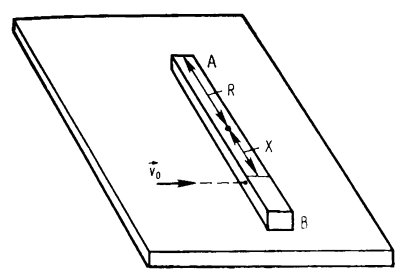Problem Picture. If not visible go here -----> https://i.imgur.com/VjzHXED.png

Will a velocity directed in opposition to $v_0$ be imparted to end A at the first moment after collision ? Why ? Why not ?

Kaustubh Miglani solved it first along with a Nice Solution(incomplete) then Rajdeep Dhingra posted the complete Solution

- 5 years, 4 months ago

Log in to reply

Solution to problem 15:

When the bullet enters the rod it will de-accelerate This means that it will undergo a force in opposite direction to its velocity with -ma According to newtons third law of motion,every action has equal and opp reaction.So ,the bullet too will exert a force on the rod In this case Torque comes into play As the Torque is given by $frsin(\theta)$ where $\theta$ is angle between force and r vector In this case none of them is zero ,So it will produce a rotational effect in the direction of force. So ,if it rotates in direction of force A will move in direction opposite to $v_0$. But if $x>l/2$ the bullet escapes the rod with no rotational effect So for $0 THE BULLET escapes the rod without any effect.(Rotational)

- 5 years, 4 months ago

Log in to reply

Solution Of Problem 15 :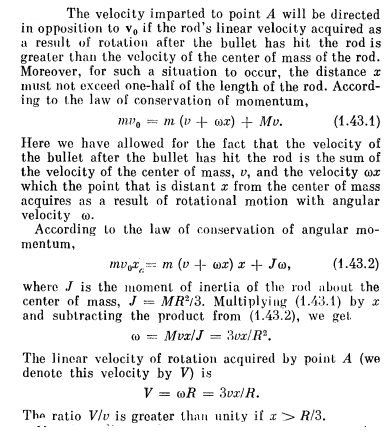- 5 years, 4 months ago

Log in to reply

Problem 16:

A string with a bob is attached to a surface and lies vertically .It is given a horizontal velocity $v_0$ and it moves in a circle for some time.However after some time the string goes slack at a point and bob is unable to touch the top. Instead the bob,under action of gravity strikes the point where the string is attached.Take the length of the string as L. Find the velocity(s) for which the above conditions are satisfied.

Surya Prakash has solved it along with a Great Solution

- 5 years, 4 months ago

Log in to reply

Solution To Problem 16 :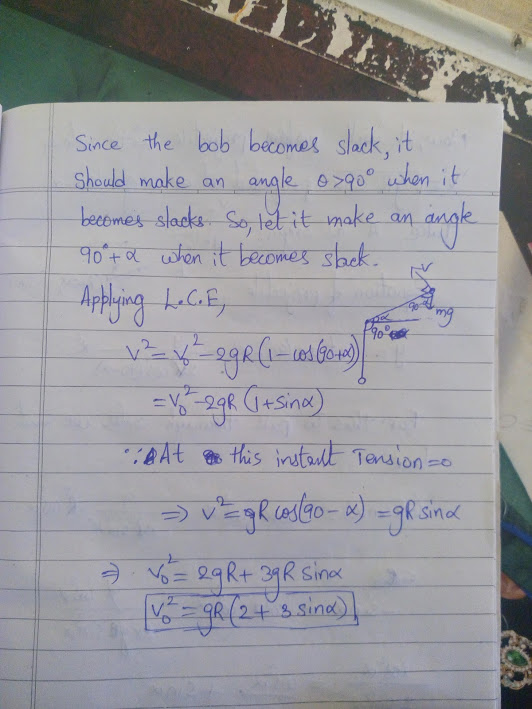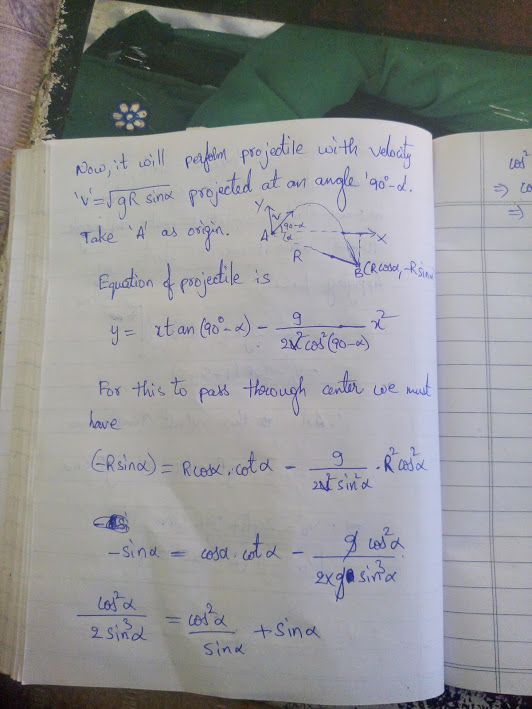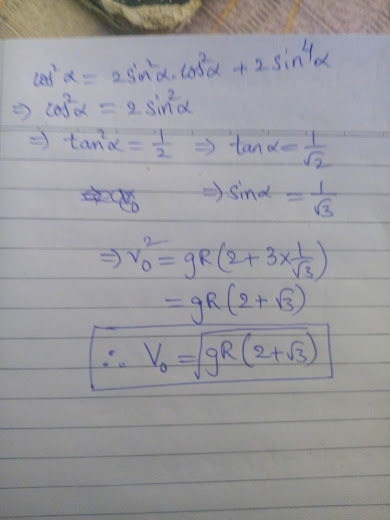- 5 years, 4 months ago

Log in to reply

Is the contest over??

- 5 years, 1 month ago

Log in to reply

Can we revive this?

- 2 years, 3 months ago

Log in to reply

Please?

- 2 years ago

Log in to reply

Lol more like Indian people brilliant.org hangout

- 1 year, 1 month ago

Log in to reply

×

Problem Loading...

Note Loading...

Set Loading...